# Nuts and Bolts of Reinforcement Learning: Introduction to Temporal Difference (TD) Learning

## Introduction

Q-learning became a household name in data science when DeepMind came up with an algorithm that reached superhuman levels on ATARI games. It’s one of the core components of reinforcement learning (RL). I regularly come across Q-learning whenever I’m reading up about RL.

But what does Q-learning have to do with our topic of temporal difference learning? Let me take an example to give an intuition of what temporal difference learning is about.

Rajesh is planning to travel to Jaipur from Delhi in his car. A quick check on Google Maps shows him an estimated 5 hour journey. Unfortunately, there is an unexpected delay due to a roadblock (anyone who has taken a long journey can relate to this!). The estimated arrival time for Rajesh now jumps up to 5 hours 30 minutes.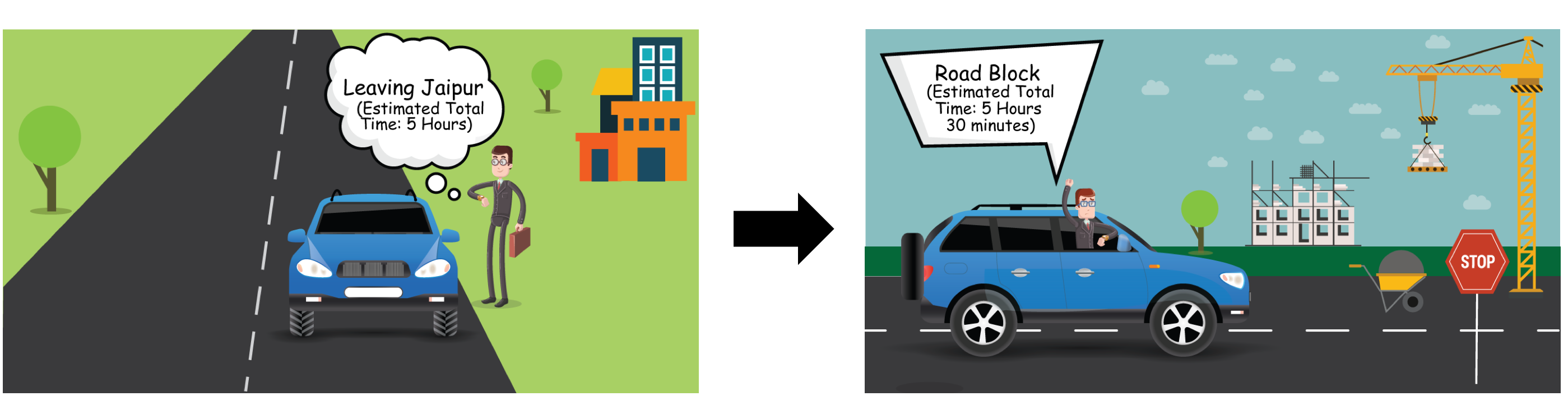Halfway through the journey, he finds a bypass that reduces his arrival time. So overall, his journey from Delhi to Jaipur takes 5 hours 10 minutes.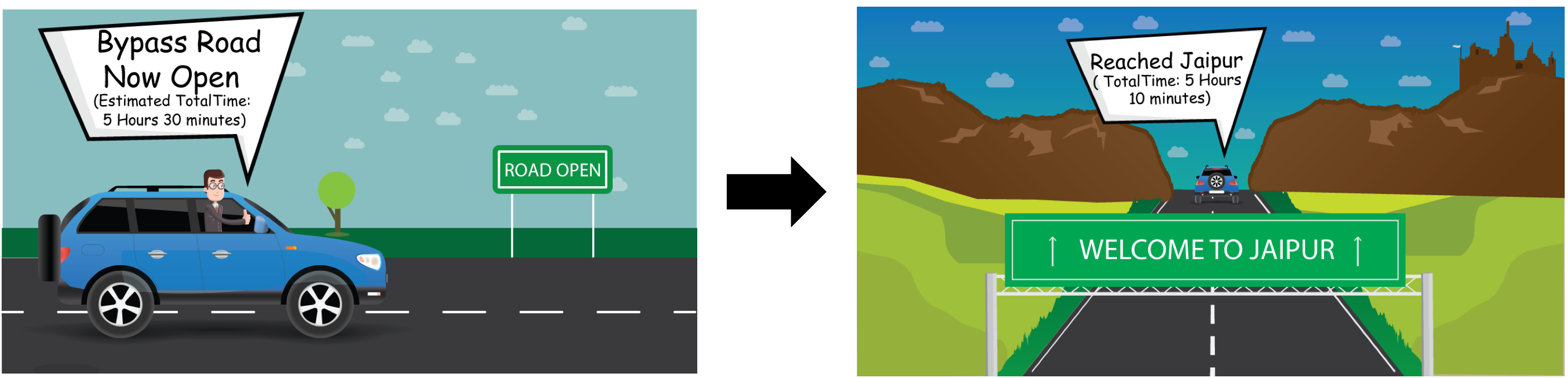Did you notice how Rajesh’s arrival time kept changing and updating based on different episodes? This, in a nutshell, illustrates the temporal difference learning concept. In this article, I will introduce you to this algorithm and it’s components in detail, including how Q-learning fits into the picture. We’ll also pick up a case study and solve it in Python.

This is one of the most intriguing reinforcement learning concepts so let’s have fun learning this!

## Table of Contents

• Introduction to Temporal Difference (TD) Learning
• TD Prediction
• TD Control
• Off-Policy vs On-Policy Learning
• Q-learning: Off-Policy Temporal Difference Learning
• SARSA: On-Policy Temporal Difference Learning
• Case Study: Taxi Scheduling using Q-learning in Python

## Introduction to Temporal Difference (TD) Learning

We developed an intuition for temporal difference learning in the introduction. Now let’s understand it in a bit more detail.

In my previous article on Monte Carlo Learning, we learned how to use it for solving the Markov Decision Process (MDP) when the model dynamics of the environment are not known in advance. So why don’t we just use that instead of TD?

Well, although Monte Carlo learning provides an efficient and simple approach for model-free learning, there are some limitations to it. It can be applied only for episodic tasks.

An episodic task lasts a finite amount of time. For example, playing a single game of Chess is an episodic task, which you win or lose. If an episode is very long (which it is in many cases), then we have to wait a long time to compute value functions.

Temporal difference (TD) learning, which is a model-free learning algorithm, has two important properties:

• It doesn’t require the model dynamics to be known in advance
• It can be applied for non-episodic tasks as well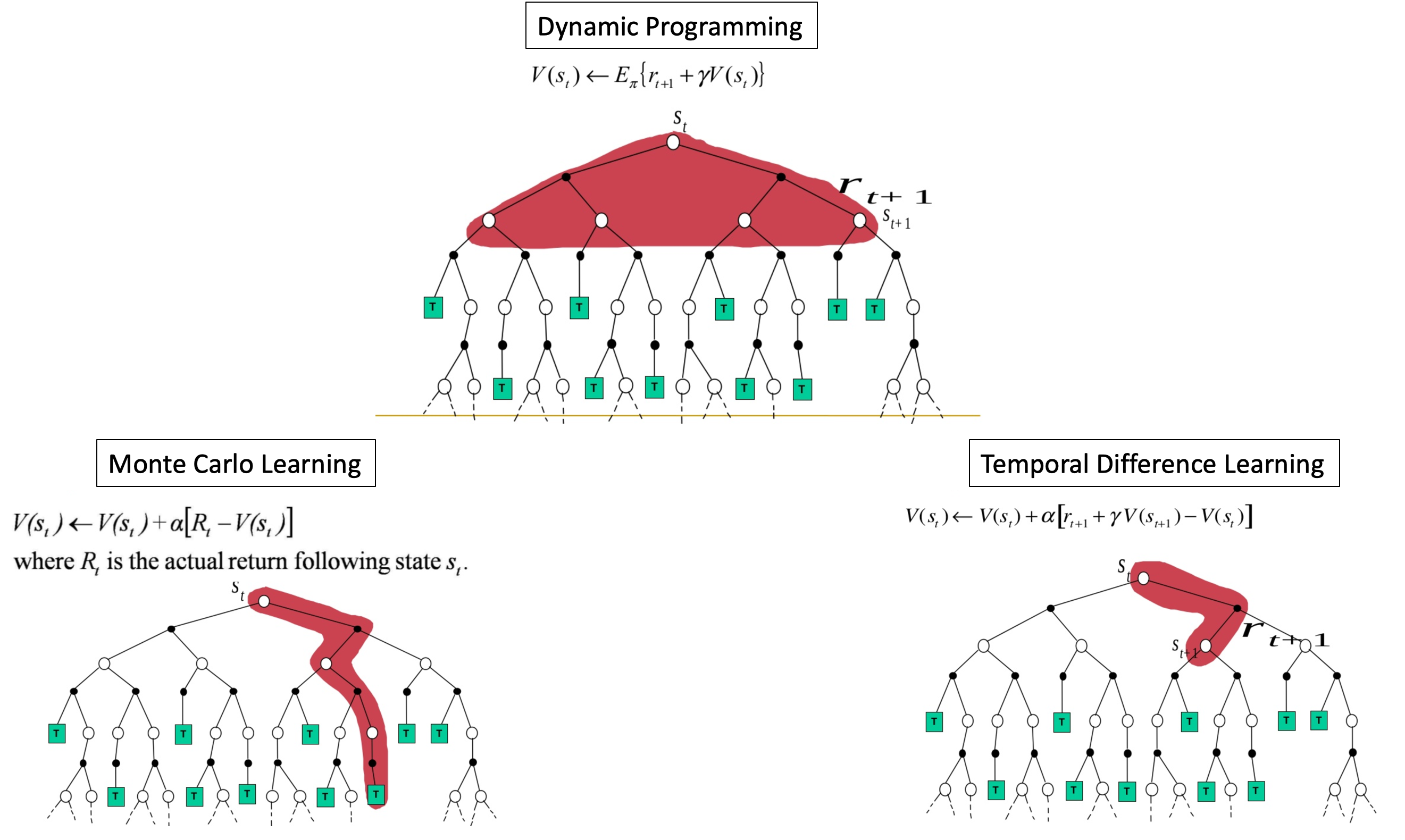The TD learning algorithm was introduced by the great Richard Sutton in 1988. The algorithm takes the benefits of both the Monte Carlo method and dynamic programming (DP) into account:

• Like the Monte Carlo method, it doesn’t require model dynamics, and
• Like dynamic programming, it doesn’t need to wait until the end of the episode to make an estimate of the value function

Instead, temporal difference learning approximates the current estimate based on the previously learned estimate. This approach is also called bootstrapping.

### Getting the Intuition Behind TD Prediction

We try to predict the state values in temporal difference learning, much like we did in Monte Carlo prediction and dynamic programming prediction. In Monte Carlo prediction, we estimate the value function by simply taking the mean return for each state whereas in Dynamic Programming and TD learning, we update the value of a previous state by the current state. But TD learning does not need model of the environment unlike DP.

How can we do this? TD learning using something called a TD update rule for updating the value of a state: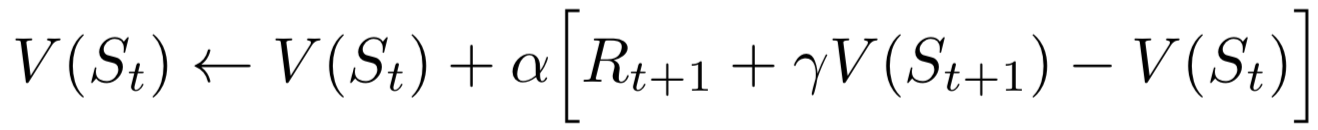value of a previous state = value of previous state + learning_rate * (reward + discount_factor(value of current state) – value of previous state)

What does this equation actually mean?

This is the difference between the actual reward (r + Gamma * V(s’)) and the expected reward V(s) multiplied by the learning rate alpha.

What does the learning rate signify?

The learning rate, also called step size, is useful for convergence.

Since we take the difference between the actual and predicted values, this is like an error. We can call it a TD error.  Notice that the TD error at each time is the error in the estimate made at that time. Because the TD error depends on the next state and next reward, it is not actually available until one timestep later. Iteratively, we will try to minimize this error.

#### Understanding TD Prediction using the Frozen Lake Example

Let us understand TD prediction with the frozen lake example. The frozen lake environment is shown next. First, we will initialize the value function as 0, as in V(S) as 0 for all states, as shown in the following state-value diagram:

##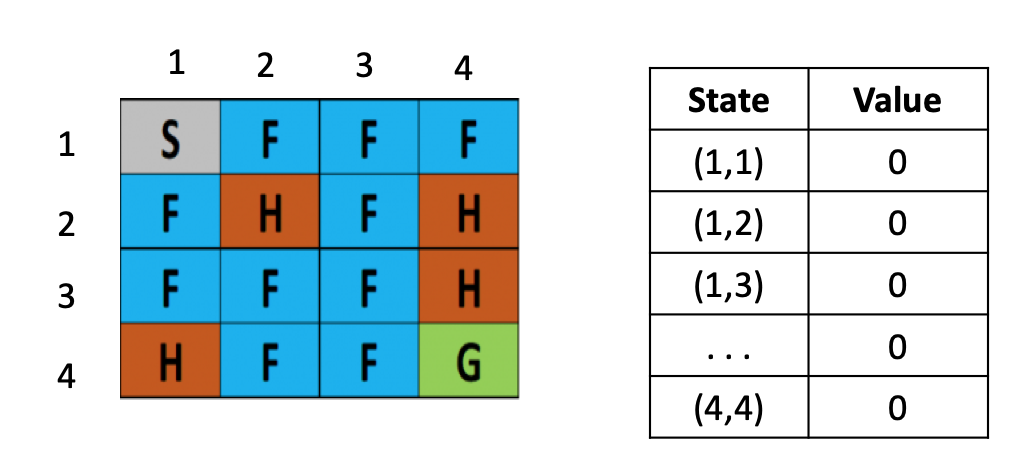Say we are in a starting state (s) (1,1) and we take an action right and move to the next state (s’) (1,2) and receive a reward (r) as -0.4.

How can we update the value of the state using this information? Recall the TD update equation:Let us consider the learning rate (α) as 0.1 and the discount factor () as 0.5; we know that the value of the state (1,1), as in v(s), is 0 and the value of the next state (1,2), as in V(s’), is also 0. The reward (r) we obtained is -0.3. We substitute this in the TD rule as follows:

V(s) = 0 + 0.1 [ -0.4 + 0.5 (0)-0]

V(s) = – 0.04

So, we update the value for the state (1,1) as -0.04 in the value table, as shown in the following diagram: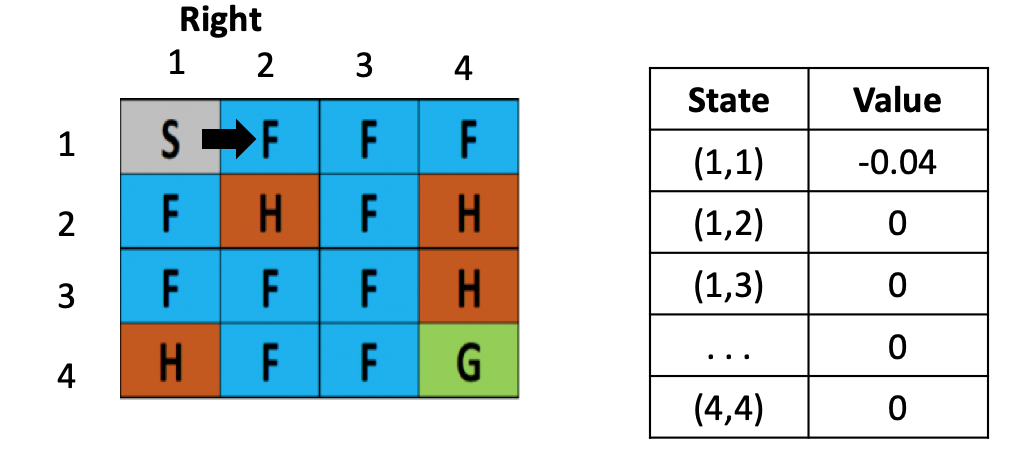Now that we are in the state (s) as (1,2), we take an action right and move to the next state (s’) (1,3) and receive a reward (r) -0.4. How do we update the value of the state (1, 2) now? We will substitute the values in the TD update equation:

V(s) = 0 + 0.1 [ -0.4 + 0.5(0)-0 ]

V(s) = -0.04

Go ahead and update the value of state (1,2) as -0.04 in the value table: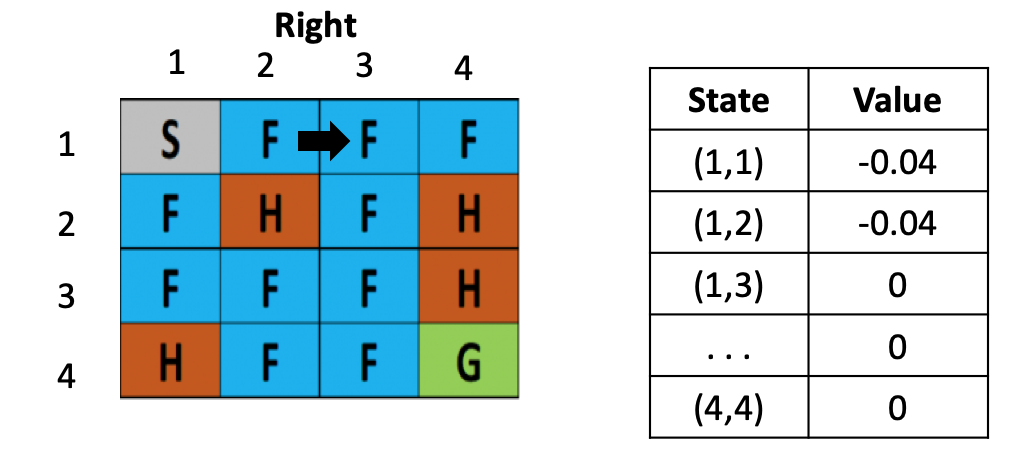With me so far? We are now in the state (s) (1,3). Let’s take an action left.

We again go back to that state (s’) (1,2) and we receive a reward (r) -0.3. Here, the value of the state (1,3) is 0 and the value of the next state (1,2) is -0.03 in the value table. Now we can update the value of state (1,3) as follows:

V(s) = 0 +0.1 [ -0.4 + 0.5 (-0.04)-0) ]

V(s) = 0.1[-0.42]

V(s) = -0.042

You know what to do now. Update the value of state (1,3) as -0.042 in the value table: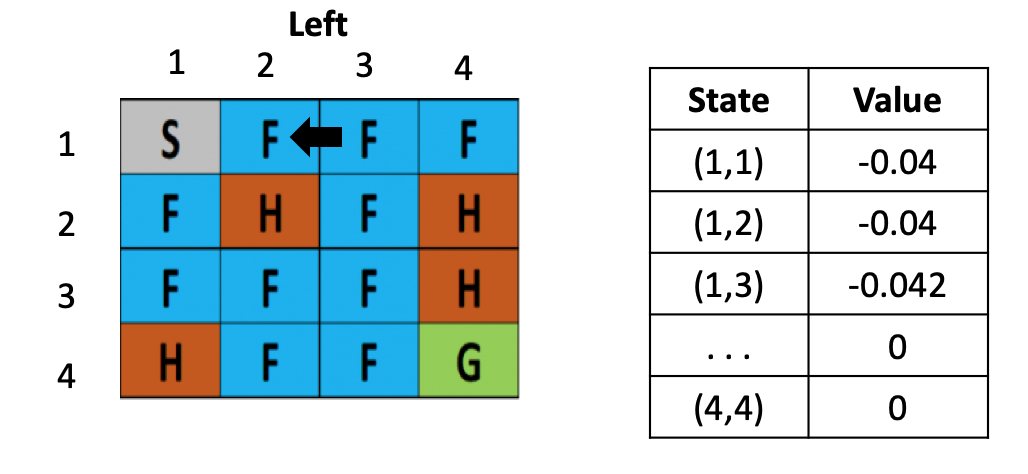We update the value of all the states in a similar fashion using the TD update rule. To summarize, the steps involved in the TD prediction algorithm are:

1. First, initialize V(S) to 0 or some arbitrary value
2. Then, begin the episode. For every step in the episode, perform an action A in the state S and receive a reward R and move to the next state (s’)
3. Update the value of the previous state using the TD update rule
4. Repeat steps 2 and 3 until we reach the terminal state

### Understanding Temporal Differencing Control

In Temporal Difference prediction, we estimated the value function. In TD control, we optimize the value function. There are two kinds of algorithms we use for TD Control:

• Off-policy learning algorithm: Q-learning
• On-policy learning algorithm: SARSA

Off-Policy vs On-Policy

What’s the difference between off-policy and on-policy learning? The answer lies in their names:

• Off-policy learning: The agent learns about policy π from experience sampled from another policy µ
• On-policy learning: The agent learns about policy π from experience sampled from the same policy π

Let me break this down in the form of an example. Let’s say you joined a new firm as a data scientist. In this scenario, you can equate on-policy learning as learning on the job. You’ll be trying different things and learning only from your own experience.

Off-policy learning would be where you have full access to the actions of another employee. All you do in this scenario is learn from that employee’s experience and not repeat something that the employee has failed at.

## Q-learning

Q-learning is a very popular and widely used off-policy TD control algorithm.

In Q learning, our concern is the state-action value pair—the effect of performing an action a in the state s. This tells us how good an action is for the agent at a particular state (Q(s,a)), rather than looking only at how good it is to be in that state (V(s))

We will update the Q value based on the following equation: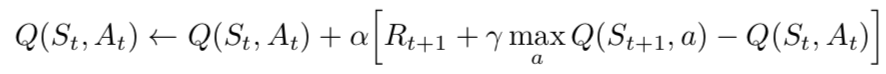Why is Q-learning considered as an off-policy technique? This is because it updates its Q-values using the Q-value of the next state 𝑠′ and the greedy action 𝑎′. In other words, it estimates the return (total discounted future reward) for state-action pairs assuming a greedy policy (maxQ(s’a’)) was followed despite the fact that it’s not following a greedy policy!

The above equation is similar to the TD prediction update rule with a subtle difference. Below are the steps involved in Q-learning (I want you to notice the difference here):

1. First, initialize the Q function to some arbitrary value
2. Take an action from a state using epsilon-greedy policy () and move it to the new state
3. Update the Q value of a previous state by following the update rule
4. Repeat steps 2 and 3 till we reach the terminal state

Now, let’s go back to our example of Frozen Lake. Let us say we are in a state (3,2) and have two actions (left and right). Refer to the below figure: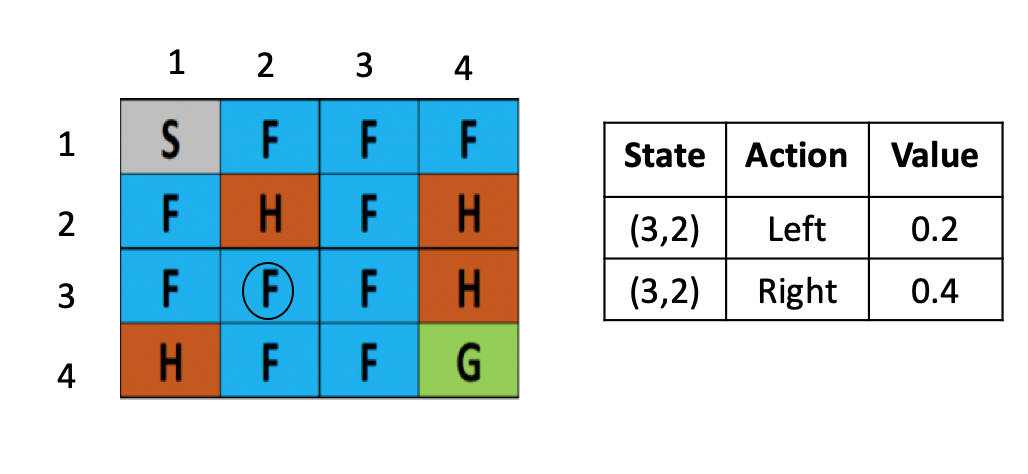We select an action using the epsilon-greedy policy in Q-learning. We either explore a new action with the probability epsilon or we select the best action with a probability 1 – epsilon. Suppose we select a probability epsilon and select a particular action (moving down):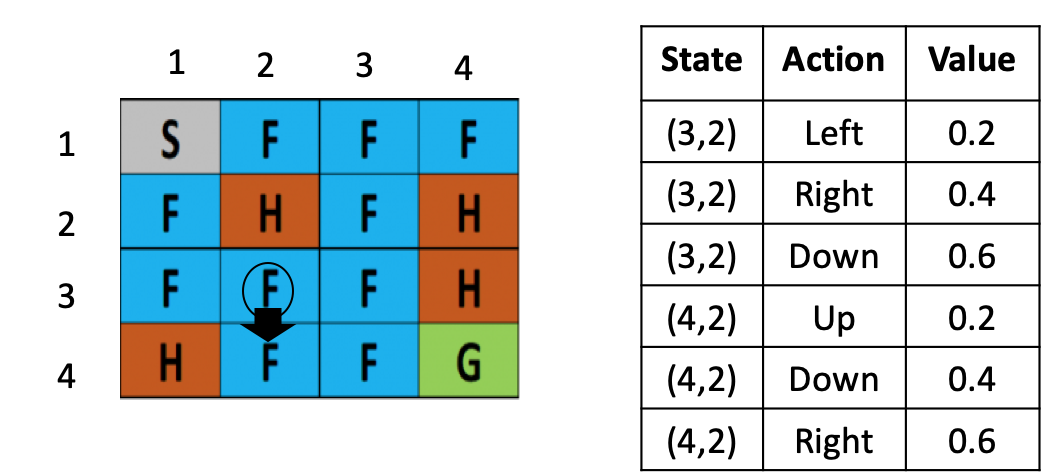We have performed a downward action in the state (3,2) and reached a new state (4,2) using the epsilon-greedy policy. How do we update the value of the previous state (3,2) using our update rule? It’s pretty straightforward!

Let us consider alpha as 0.1, the discount factor as 1 and reward as 0.4:Q( (3,2) down) = Q( (3,2) down ) + 0.1 ( 0.4 + 1 max [Q( (4,2) action) ]- Q( (3,2), down)

We can say the value of a state (3,2) with downward action is 0.6 in the Q table. What is max Q ( (4,2), action) for the state (4,2)?

We have explored three actions (up, down, and right) so we will take the maximum value based on these actions only. There is no exploration involved here – this is a straightforward greedy policy.

Based on the previous Q table, we can plug in the values:

Q( (3,2), down) = 0.6 + 0.1 ( 0.4 + 1 * max [0.2, 0.4, 0.6] – 0.6)

Q( (3,2), down) = 0.64

So, we update the value of Q ((3,2), down) to 0.64.

Now, we are in the (4,2) state. What action should we perform? Based on the epsilon-greedy policy, we can either explore a new action with a probability epsilon, or select the best action with a probability 1 – epsilon. Let us say we select the latter option. So, in (4,2), the action right has a maximum value and that’s what we’ll select: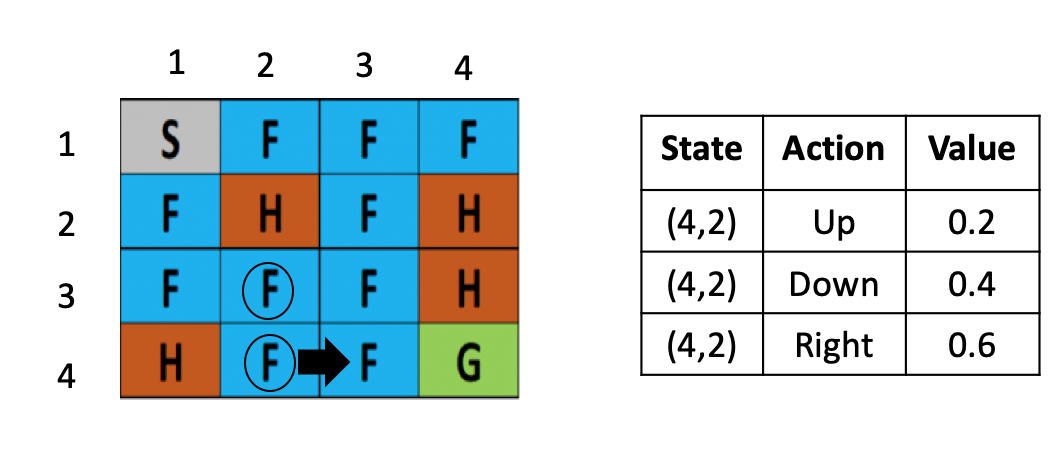Right, we are have moved to the state (4,3). Things are shaping up nicely so far. But wait – how do we update the value of the previous state?

Q( (4,2), right) = Q( (4,2), right ) + 0.1 ( 0.4 + 1*max [Q( (4,3) action) ]- Q( (4,2), right)

If you look at the Q table that follows, we have explored only two actions (up and down) for the state (4,3). So, we will take a maximum value based only on these actions (we will not perform an epsilon-greedy policy here; we simply select the action which has maximum value):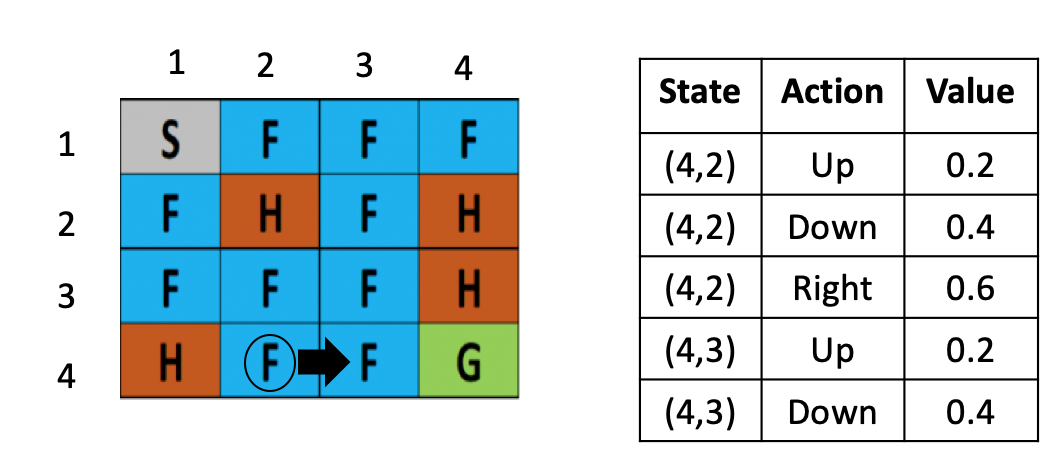Q ( (4,2), right) = Q((4,2),right) + 0.1 (0.4 + 1 max [ (Q (4,3), up) , ( Q(4,3),down) ] – Q ((4,2), right)

Q ( (4,2), right) = 0.6 + 0.1 (0.4 + 1 max [ 0.2,0.4] – 0.8)

= 0.6 + 0.1 (0.4 + 1(0.4) – 0.6)

= 0.62

Awesome! We’ll update the value of the state Q((4,2), right) to 0.62.

This is how we get the state-action values in Q-learning. We use the epsilon-greedy policy to decide what action to take, and simply pick the maximum action while updating the Q value.

### SARSA

State-Action-Reward-State-Action (SARSA) is an on-policy TD control algorithm. Similar to what we did in Q-learning, we focus on state-action value instead of a state-value pair. In SARSA, we update the Q value based on the below update rule: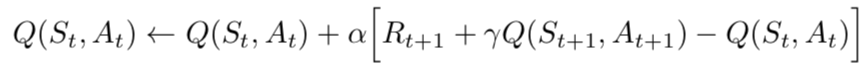You might have noticed that there is no max Q(s’,a’) (unlike Q-learning). Here, it is simply Q(s’,a’). You’ll understand this when you go through the below SARSA steps:

1. First, initialize the Q values to some arbitrary values
2. Select an action by the epsilon-greedy policy () and move from one state to another
3. Update the Q value in the previous state by following the update rule, where a’ is the action selected by an epsilon-greedy policy ()
4. Now we’ll understand the algorithm step-by-step

Let us consider the same frozen lake example. Suppose we are in state (4,2). We decide the action based on the epsilon-greedy policy. Let’s say we use a probability 1 – epsilon and select the best action (moving right):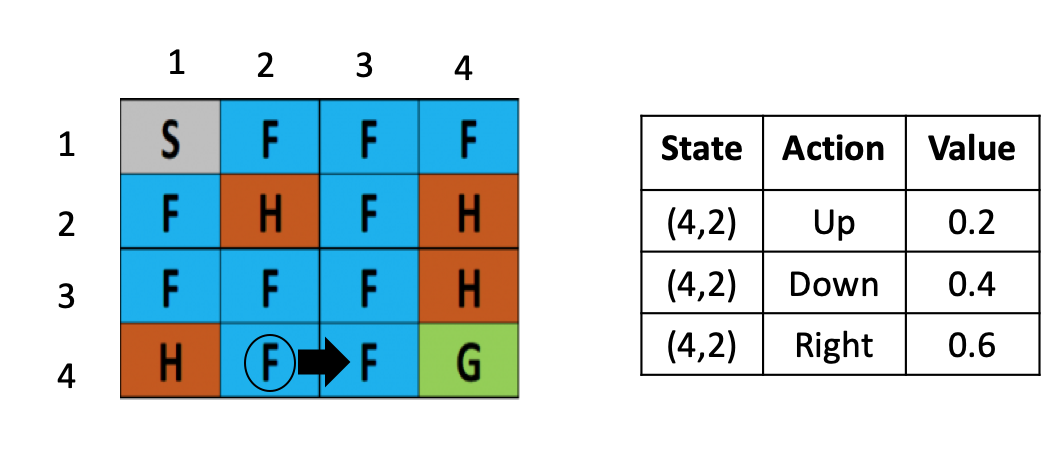We land in the state (4,3). How do we update the value of the previous state (4,2)? I’ll pull up the above equation and plug in the values. Let us consider the alpha as 0.1, the reward as 0.4, and discount factor as 1:Q( (4,2), right) = Q( (4,2),right) + 0.1 ( 0.4 + 1 *Q( (4,3), action)) – Q((4,2) , right)

How do we choose the value for Q ((4,3), action)? We can’t just pick up max ( Q(4,3), action) like we did in Q-learning. In SARSA, we use the epsilon-greedy policy. Look at the Q table I’ve shown below. In state (4,3), we have explored two actions:We either explore with a probability epsilon or exploit with a probability 1 – epsilon. Let’s say we select the former option and explore a new action (moving right):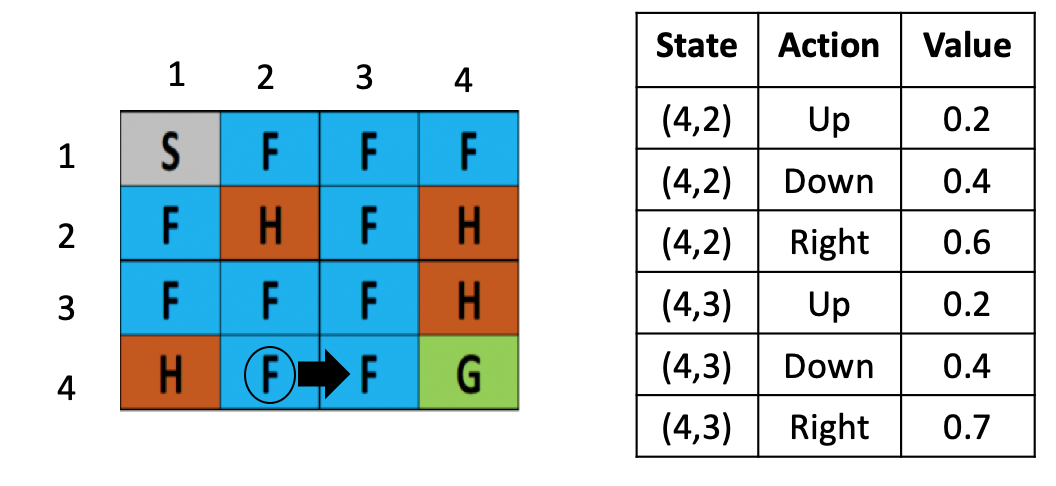Q ( (4,2), right) = Q((4,2),right) + 0.1 (0.4 + 1 (Q (4,3), right) – Q ((4,2), right )

Q ( (4,2), right) = 0.6 + 0.1 (0.4 + 1(0.7) – 0.6)

Q ( (4,2), right) = 0.65

And that’s how we get the state-action values in SARSA. We take the action using the epsilon-greedy policy and pick the action using the epsilon-greedy policy updating the Q value.

## Case Study in Python: Taxi Scheduling using Q-learning

We’ve finally come to the implementation part of our article! Time to fire up our Jupyter notebook and get our hands dirty. We will demonstrate Q-learning in action with an OpenAI Gym environment called Taxi-V2.

The aim of this project – our agent must pick up the passenger at one location and drop him off at the destination/goal as fast as possible.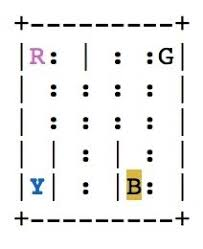R, G, Y and B in the above image are the 4 locations we will consider for our project. A few pointers:

• You receive +20 points for a successful drop-off
• Lose 1 point for every timestep the agent takes
• There is also a 10-point penalty for illegal pick-up and drop-off actions (if you don’t drop the passenger in one of the 3 other locations)

Let’s begin!

### Step 1: Import dependencies & Create the Environment

```import numpy as np
import gym
import random

env = gym.make("Taxi-v2")
env.render()```

### Step 2: Create the Q-table and initialize it

• We’ll create our Q-table to know how many rows (states) and columns (actions) we need. We need to calculate the action_size and the state_size
• OpenAI Gym provides us a way to do that: `env.action_space.n` and `env.observation_space.n`
```action_size = env.action_space.n
print("Action size ", action_size)

state_size = env.observation_space.n
print("State size ", state_size)``````qtable = np.zeros((state_size, action_size))
print(qtable)```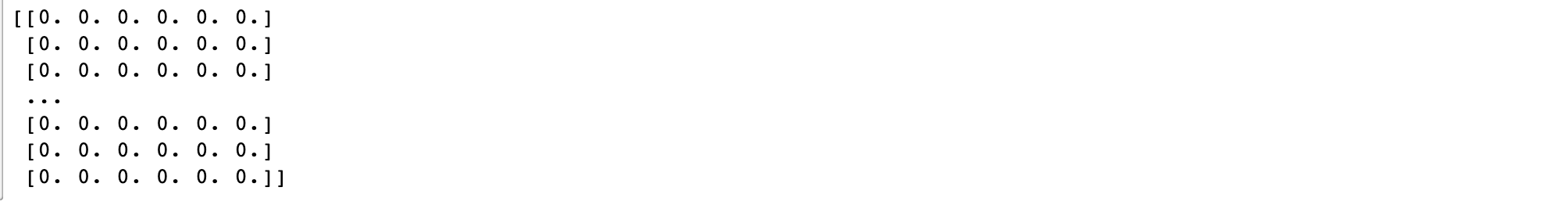### Step 3: Setting Hyperparameters

We’ll specify the hyperparameters at this step:

```total_episodes = 50000 # Total episodes
total_test_episodes = 100 # Total test episodes
max_steps = 99 # Max steps per episode

learning_rate = 0.7 # Learning rate
gamma = 0.618 # Discounting rate

# Exploration parameters
epsilon = 1.0 # Exploration rate
max_epsilon = 1.0 # Exploration probability at start
min_epsilon = 0.01 # Minimum exploration probability
decay_rate = 0.01 # Exponential decay rate for exploration prob```

### Step 4: The Q-learning Algorithm

Time to put our theoretical learning to the test! Let’s implement the Q-learning algorithm in Python:

```# 2 For life or until learning is stopped
for episode in range(total_episodes):
# Reset the environment
state = env.reset()
step = 0
done = False

for step in range(max_steps):
# 3. Choose an action a in the current world state (s)
## First we randomize a number
exp_exp_tradeoff = random.uniform(0,1)

## If this number > greater than epsilon --> exploitation (taking the biggest Q value for this state)
if exp_exp_tradeoff > epsilon:
action = np.argmax(qtable[state,:])

# Else doing a random choice --> exploration
else:
action = env.action_space.sample()

# Take the action (a) and observe the outcome state(s') and reward (r)
new_state, reward, done, info = env.step(action)

# Update Q(s,a):= Q(s,a) + lr [R(s,a) + gamma * max Q(s',a') - Q(s,a)]
qtable[state, action] = qtable[state, action] + learning_rate * (reward + gamma *
np.max(qtable[new_state, :]) - qtable[state, action])

# Our new state is state
state = new_state

# If done : finish episode
if done == True:
break

# Reduce epsilon (because we need less and less exploration)
epsilon = min_epsilon + (max_epsilon - min_epsilon)*np.exp(-decay_rate*episode)

```

### Step 5: Use our Q-table to play Taxi!

After 50,000 episodes, our Q-table can be used as a “cheatsheet” to play the role of the Taxi.

```env.reset()
rewards = []

for episode in range(total_test_episodes):
state = env.reset()
step = 0
done = False
total_rewards = 0
#print("****************************************************")
#print("EPISODE ", episode)

for step in range(max_steps):
# UNCOMMENT IT IF YOU WANT TO SEE OUR AGENT PLAYING
# env.render()
# Take the action (index) that have the maximum expected future reward given that state
action = np.argmax(qtable[state,:])

new_state, reward, done, info = env.step(action)

total_rewards += reward

if done:
rewards.append(total_rewards)
#print ("Score", total_rewards)
break
state = new_state
env.close()
print ("Score over time: " + str(sum(rewards)/total_test_episodes))```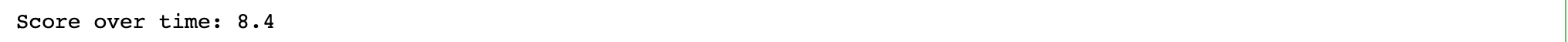As you can see the overall score is 8.4. This basically says that on an average the agent is scoring 8.4 points each episode after learning from 50000 episodes. Try increasing the maximum episodes and you will see that the score on the test episodes will increase.

## End Notes

TD Learning is the most widely used reinforcement learning method today. This is probably due to their great simplicity: they can be applied online, with a minimal amount of computation, to experience generated from interaction with an environment; they can be expressed nearly completely by single equations that can be implemented with small computer programs.

There are extensions to TD learning which makes them slightly more complicated and significantly more powerful. The special cases of TD methods introduced in the article should rightly be called one-step, tabular, model-free TD methods. We can extend them to n-step forms (a link to Monte Carlo methods). We can also extend them to various forms of function approximation rather than tables (a link to deep learning and artificial neural networks) which is where Deep Q Learning comes into the picture.

Here’s a challenge for the readers. I encourage everyone to use the provided implementation to implement SARSA algorithm. Let me know how it works out and share your code in the comment section.

You can also read this article on Analytics Vidhya's Android APP### 3 Comments

•neha agarwal says:

Excellent inforamation for easily understandable even for common man

•Sadia siddiqui says:

Very well explained. Thank you.

•Gurpreet Singh says:

Great explanation. Thank you.Download ResourceDownload Resource